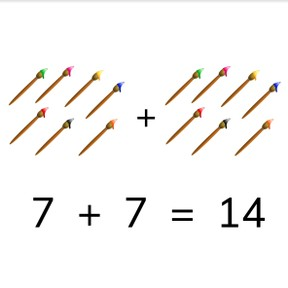8,000 schools use Gynzy92,000 teachers use Gynzy1,600,000 students use Gynzy

## General

Students learn to add doubles to a total of 20.

1.OA.C

## Relevance

It is important that students are able to recognize doubles and are able to calculate their total to help improve ease of calculation.

## Introduction

The interactive whiteboard shows three number sequences under twenty. Students determine if numbers are in order or out of order. They can erase the grey box to show the answer.

## Development

Explain that when you add two numbers that are the same, you double the number. You can show this by counting the paintbrushes. The students calculate using the images on the interactive whiteboard. They can count the objects. With the eggs and pencils, ask the students to come up with the addition problem represented by the objects, before having them solve those addition problems. Next show students how to solve an abstract addition problem, without the visual support and only showing numbers. Do this by adding the weights. Next you can discuss adding all the doubles to 10 one by one. The students then calculate a few problems without visual support. Finally they are asked to drag the totals to the addition problems shown.

Check that students understand adding doubles to a total of 20 by asking the following questions:
- What do you do if you double a number?
- What is the total of 8+8?

## Guided practice

Students first practice adding doubles with visual support. Next they must add doubles without visual support.

## Closing

Repeat the goal and discuss why it is useful to be able to add doubles. The interactive whiteboard shows an addition problem. Two students give an answer, and the class must determine which of the two students shown is correct.

## Teaching tips

Students who have difficulty with this goal can be given blocks or manipulatives to use in calculation. Have them make groups of blocks to represent each addend, and then count the blocks together.

## Instruction materials

Optional: blocks or manipulatives

### The online teaching platform for interactive whiteboards and displays in schools

• Save time building lessons

• Manage the classroom more efficiently

• Increase student engagement